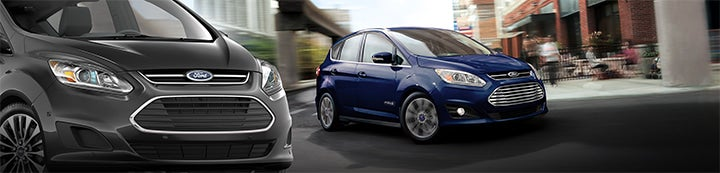1 - 4 of 4 Posts

#### Denzil Cootlar

·
##### Registered
Joined
·
7 Posts
Discussion Starter · ·
Received my new CMax 2.0 Titanium on 1-8-08 - Immediately had to book it in for a safety recall over the possibility of the power steering hose splitting causing fluid to hit hot engine parts resulting in an engine fire. Seems that a faulty batch of hoses have been used. I was lucky to get an immediate notification from my supplying dealer as the notification arrived on the day of delivery.
If anyone has the new shape I suggest you contact you local Ford dealer to see if your's is effected by the recall. Took half an hour today for the dealer to read the part serial number to check if it was part of the faulty batch.

·
Joined
·
3,966 Posts

·
Joined
·
201 Posts

#### Richard G

·
Joined
·
3,966 Posts
I'd looked for Focus C Max which is why

Here is an extended production Year and Month (digits 11 and 12)

|________|JAN|FEB|MAR|APR|MAY|JUN|JUL|AUG|SEP|OCT|NOV|DEC|
|_A_|1980|_B_|_R_|_A_|_G_|_C_|_K_|_D_|_E_|_L_|_Y_|_S_|_T_|
|_B_|1981|_J_|_U_|_M_|_P_|_B_|_R_|_A_|_G_|_C_|_K_|_D_|_E_|
|_C_|1982|_L_|_Y_|_S_|_T_|_J_|_U_|_M_|_P_|_B_|_R_|_A_|_G_|
|_D_|1983|_C_|_K_|_D_|_E_|_L_|_Y_|_S_|_T_|_J_|_U_|_M_|_P_|
|_E_|1984|_B_|_R_|_A_|_G_|_C_|_K_|_D_|_E_|_L_|_Y_|_S_|_T_|
|_F_|1985|_J_|_U_|_M_|_P_|_B_|_R_|_A_|_G_|_C_|_K_|_D_|_E_|
|_G_|1986|_L_|_Y_|_S_|_T_|_J_|_U_|_M_|_P_|_B_|_R_|_A_|_G_|
|_H_|1987|_C_|_K_|_D_|_E_|_L_|_Y_|_S_|_T_|_J_|_U_|_M_|_P_|
|_J_|1988|_B_|_R_|_A_|_G_|_C_|_K_|_D_|_E_|_L_|_Y_|_S_|_T_|
|_K_|1989|_J_|_U_|_M_|_P_|_B_|_R_|_A_|_G_|_C_|_K_|_D_|_E_|
|_L_|1990|_L_|_Y_|_S_|_T_|_J_|_U_|_M_|_P_|_B_|_R_|_A_|_G_|
|_M_|1991|_C_|_K_|_D_|_E_|_L_|_Y_|_S_|_T_|_J_|_U_|_M_|_P_|
|_N_|1992|_B_|_R_|_A_|_G_|_C_|_K_|_D_|_E_|_L_|_Y_|_S_|_T_|
|_P_|1993|_J_|_U_|_M_|_P_|_B_|_R_|_A_|_G_|_C_|_K_|_D_|_E_|
|_R_|1994|_L_|_Y_|_S_|_T_|_J_|_U_|_M_|_P_|_B_|_R_|_A_|_G_|
|_S_|1995|_C_|_K_|_D_|_E_|_L_|_Y_|_S_|_T_|_J_|_U_|_M_|_P_|
|_T_|1996|_B_|_R_|_A_|_G_|_C_|_K_|_D_|_E_|_L_|_Y_|_S_|_T_|
|_V_|1997|_J_|_U_|_M_|_P_|_B_|_R_|_A_|_G_|_C_|_K_|_D_|_E_|
|_W_|1998|_L_|_Y_|_S_|_T_|_J_|_U_|_M_|_P_|_B_|_R_|_A_|_G_|
|_X_|1999|_C_|_K_|_D_|_E_|_L_|_Y_|_S_|_T_|_J_|_U_|_M_|_P_|
|_Y_|2000|_B_|_R_|_A_|_G_|_C_|_K_|_D_|_E_|_L_|_Y_|_S_|_T_|
|_1_|2001|_J_|_U_|_M_|_P_|_B_|_R_|_A_|_G_|_C_|_K_|_D_|_E_|
|_2_|2002|_L_|_Y_|_S_|_T_|_J_|_U_|_M_|_P_|_B_|_R_|_A_|_G_|
|_3_|2003|_C_|_K_|_D_|_E_|_L_|_Y_|_S_|_T_|_J_|_U_|_M_|_P_|
|_4_|2004|_B_|_R_|_A_|_G_|_C_|_K_|_D_|_E_|_L_|_Y_|_S_|_T_|
|_5_|2005|_J_|_U_|_M_|_P_|_B_|_R_|_A_|_G_|_C_|_K_|_D_|_E_|
|_6_|2006|_L_|_Y_|_S_|_T_|_J_|_U_|_M_|_P_|_B_|_R_|_A_|_G_|
|_7_|2007|_C_|_K_|_D_|_E_|_L_|_Y_|_S_|_T_|_J_|_U_|_M_|_P_|
|_8_|2008|_B_|_R_|_A_|_G_|_C_|_K_|_D_|_E_|_L_|_Y_|_S_|_T_|
Production Sequence Number (digits 13 and 17)
from 10001 to 59999

The above courtesy of the "other forum" should give some idea of the relevent VIN codes

1 - 4 of 4 Posts